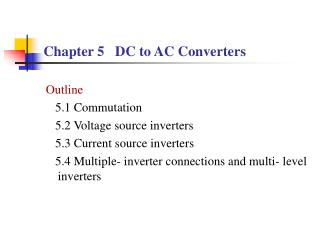DownloadDownload PresentationChapter 5 DC to AC Converters

# Chapter 5 DC to AC Converters

Download Presentation## Chapter 5 DC to AC Converters

- - - - - - - - - - - - - - - - - - - - - - - - - - - E N D - - - - - - - - - - - - - - - - - - - - - - - - - - -
##### Presentation Transcript

1. Chapter 5 DC to AC Converters Outline 5.1 Commutation 5.2 Voltage source inverters 5.3 Current source inverters 5.4 Multiple-inverter connections and multi-level inverters

2. 5.1 Commutation types • Basic operation principle of inverters A classification of inverters –Square- wave inverters (are discussed in this chapter) –PWM inverters ( will be discussed in Chapter 6) The concept of commutation

3. 4 types of commutation 1)Device commutation: Fully- controlled devices: GTO, IGBT, MOSFET 2)Line commutation: Phase- controlled rectifier,Phase- controlled AC controller, Thyristor cycloconverter 3)Load commutation 4)Forced commutation

5. (2) Forced commutation (capacitance commutation)

6. Another classification of commutations

7. 2 classes of inverters

8. 5.2 Voltage source inverter (VSI) • Features DC side is constant voltage, low impedance (voltage source, or bulk cap) AC side voltage is square wave or quasi-square wave. AC side current is determined by the load. Anti-parallel diodes are necessary to provide energy feedback path. (freewheeling diodes , feedback diodes)

9. Single-phase half bridge VSI The current conducting path is determined by the polarity of load voltage and load current. (This is true for analysis of many power electronics circuits.) The magnitude of output square- wave voltage is Ud/2.

10. Single-phase full bridge VSI Operation principle

11. Quantitative analysis Fourier series extension of output voltage Magnitude of output voltage fundamental component Effective value of output voltage fundamental component

12. Output voltage control by phase-shift

13. Three-phase VSI

14. Basic equations to obtain voltage

15. Quantitative analysis Fourier series extension of output line- to- line voltage Magnitude of output voltage (line- to- line) fundamental component Effective value of output voltage (line- to- line) fundamental component

16. 5.3Current source inverter (CSI) Features DC side is constant current , high impedance (current source, or large inductor) AC side current is quasis-quare wave. AC side voltage is determined by the load. No anti-parallel diodes are needed. sometimes series diodes are needed to block reverse voltage for other power semiconductor devices.

17. Single-phase bridge CSI Parallel Resonant Inverter

18. Three- phase self-commutated CSI

19. Three- phase force- commutated CSI